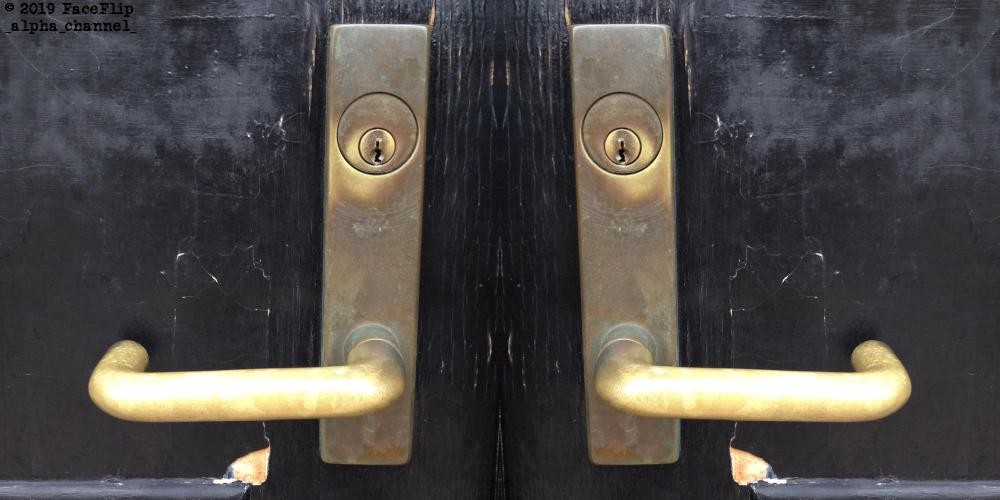C:\alpha\gmic\gmic.exe -input C:\alpha\input\test.jpg +mirror x append x -output C:\alpha\output\test_mirror_right.jpg; C:\alpha\gmic\gmic.exe -input C:\alpha\input\test.jpg mirror x +mirror x append x -output C:\alpha\output\test_mirror_left.jpg 
Now, that's a One-Liner!

Actually, it's two One-Liners!

 C:\alpha\gmic\gmic.exe -input C:\alpha\input\test.jpg +mirror x append x -output C:\alpha\output\test_mirror_right.jpg;  C:\alpha\gmic\gmic.exe -input C:\alpha\input\test.jpg mirror x +mirror x append x -output C:\alpha\output\test_mirror_left.jpg 

# G'MIC Face Flip

I've been making the rounds of the Command Line Image Processing Software (that I want to use) and this is my 'Hello World' for G'MIC.

The semicolon (;) in the above separates two lines of code (causing two separate processes to run sequentially process_one; process_two), so I will only deconstruct the second line. Furthermore, backticks ( , the space in front is required, but there can be no trailing white spaces) allow one to write a single command (or two commands separated by a semicolon) on two or more lines. And with that said, everything else required for full, complete, and total understanding of everything, everywhere at any and all times should be included in the following... or at least, everything a half decent programmer should need to understand the above code.
• C:\alpha\gmic\gmic.exe
• Invoke G'MIC
• The program is not on my System's Path.
• And as such, that's the full path to the executable on my machine.
• -input C:\alpha\input\test.jpg
• Input an image.
• The single dash (-) indicates a single image.
• Or so, I presume.
• mirror x
• Flips the image in place about the x-axis.
• The addition of this command is what differentiates the first process from the second.
• This is so I can get back-and-forth (and forth-and-back) reflected images.
• +mirror x
• Adds a flipped image onto the stack.
• So, mirror x flips the image horizontally in place.
• And +mirror x adds a horizontally flipped image to the end of the stack
• G'MIC is all about the stack.
• append x
• Stitch the images on the stack together horizontally.
• A + might be needed to stitch more than two images together.
• -output C:\alpha\output\test_mirror_left.jpg
• The single dash (-) indicates only the last image should be outputted.
• Very helpfully, +output saves every image in the stack as sequentially numbered images.
• Stupid easy.

I'm still not very good at deciphering Command Line Error Messages. But I got to tell you, this was easier than programming the same thing up in Python... which is the point of this entire _ALPHA_CHANNEL_ Project.

'Hello G'MIC' Completed!

I may now advance to the next level!

GraphicsMagick FaceFlip
G'MIC FaceFlip
PowerShell FaceFlip